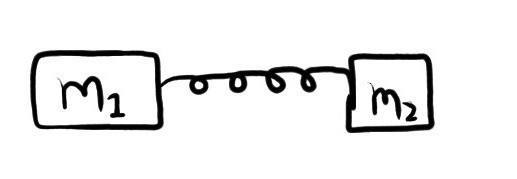# Laws of Motion Problem

## Homework Statement

Two blocks of mass m1 and m2 (m2 = 2 kg ; m1 = 3 kg)are connected with a spring moving on smooth plane. The acceleration of block of mass m2 is 2 ms-2, find acceleration of block with mass of 3kg.2. The attempt at a solution

Let external force of F be applied on Block m2.
∴ By only considering m2, we get
F - T = 6
and for block m1
T = 2a
and by newton's law of system (Fnet = m1a1+m2a2+m3a3+........)
F = 6 + 2a
But by solving these three equations, i am not getting the answer.
Plz help...........
Thanks

#### Attachments

tiny-tim
Homework Helper
hi rajumahtora!(i don't really understand the question, there seems to be something missing, but anyway …)
(m2 = 2 kg ; m1 = 3 kg)

and for block m1
T = 2a

shouldn't that be 3a ?haruspex
Homework Helper
Gold Member
2020 Award
Let external force of F be applied on Block m2.
There's no mention of an external force in the OP.
∴ By only considering m2, we get
F - T = 6
I think you're getting your m1 and m2 mixed up. m2 needs a net force of 4N.
and by newton's law of system (Fnet = m1a1+m2a2+m3a3+........)
Having considered the two masses separately, you are not going to get any additional equations by considering the whole system. If you correct your errors you will find that the whole system equation can be deduced from the other two. In fact, if there is an unknown external force, you do not have enough information to answer the question.